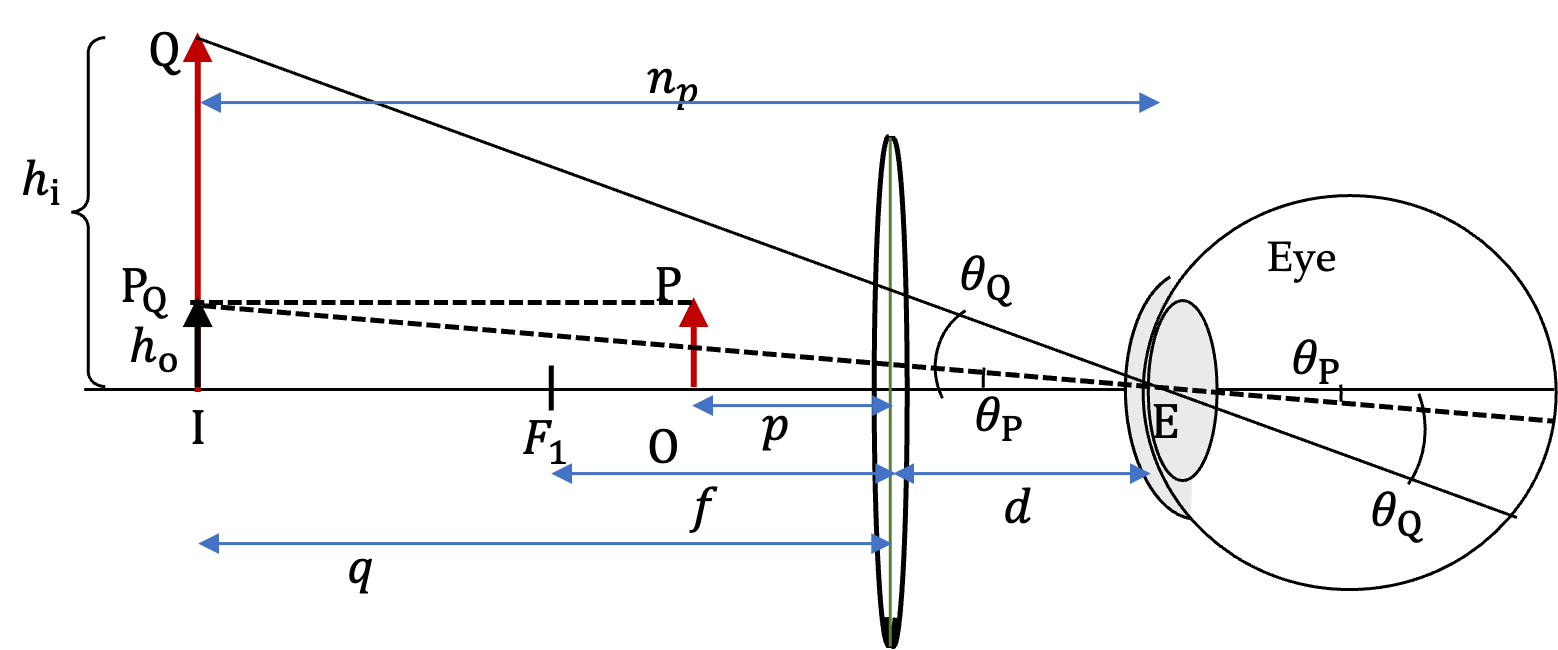## Section48.3Magnifying Glass

Recall that when an object is placed within a focal length of a convex lens its image is virtual, erect and larger than the object. Therefore, when you look at an object through a convex lens when the object is within a focal length distance behind the lens, you find it magnified. When a convex lens is used for this purpose, it is also called a magnifying glass or a simple microscope.

Our perception of magnification is based on the larger angle that the virtual image from the magnifying glass subtends at the eye. Figure 48.3.1 shows a virtual image IQ of the physical object OP. To define the angular magnification that we would perceive, we virtually move the object OP at the same distance as the virtual image IQ. This is shown as $\text{IP}_\text{Q}\text{.}$ Then, we have angle $\theta_\text{P} = \angle{\text{P}_\text{Q}\text{EI}}$ subtended at the eye by moved object and $\theta_\text{Q}=\angle\text{QEI}$ at the eye by the virtual image.

\begin{equation} \theta_\text{P} = \angle{\text{P}_\text{Q}\text{EI}},\ \ \theta_\text{Q}=\angle\text{QEI}.\tag{48.3.1} \end{equation}

The ratio of these two angles is called the angular magnification of the magnifying glass. We denote it by capital letter $M\text{.}$

\begin{equation} M = \frac{\theta_\text{Q}}{\theta_\text{P}}.\tag{48.3.2} \end{equation}

Below, we will discuss critical role that the eye plays in this magnification.Figure 48.3.1. Magnifying glass in front of an eye has the impact of “moving” the object from OP to IQ. Here $n_p$ is the distance to the near point of the eye where viewing is the clearest. Perceived magnification is given by the ratio of the angles subtended at the eye by the virtual image IQ and the “moved object” $\text{IP}_\text{Q}\text{.}$ These angles are shown as $\theta_\text{Q}$ and $\theta_\text{Q}\text{,}$ respectively. Their ratio, $\theta_\text{Q}/\theta_\text{P}$ is the angular magnification. Below, we show that $M \approx \frac{n_p+f}{f}\text{.}$

### Subsection48.3.1Role of Near Point of Eye

#### Subsubsection48.3.1.1Placing the Viewing Object

Stretch your arm and look at the tip of one of your fingers, say the index finger. They try bringing your hand closer. You will notice that the tip of the finger is clearest at about $25\text{ cm}$ from your eye. This is the nearest point of your eye. Your eye muscles are contracted in this state.

When you look through a magnifying glass, for the clearest view, you would want the image by the magnifying glass to be at your near point. So, where should you plce the object?

Refer to Figure 48.3.1 as we figure out a formula for where the object be placed for clear viewing. Let us denote the distance from your eye to the near point by $n_p\text{.}$ For a normal eye, $n_p\approx 25\text{cm}\text{.}$ Let $f$ be the focal length of the magnifying glass and $d$ the distance themagnifying glass is from your eye. This gives the image distance from the lens to be $- |n_p-d|\text{.}$

\begin{equation*} q = - |n_p-d| = -(n_p-d) \text{ since }n_p \gt d. \end{equation*}

With these, we can calculate where we should place the object for clear viewing. Let $p$ be the distance from magnifying glass to the physical object.

\begin{equation*} \frac{1}{p} = \frac{1}{f} - \left( - \frac{1}{n_p - d} \right). \end{equation*}

This gives

\begin{equation*} p = \frac{f(n_p-d)}{n_p-d+f}. \end{equation*}

Here $d \lt\lt n_p\text{,}$ and hence $d$ can be ignored.

\begin{equation} p \approx \frac{f\;n_p}{n_p+f}.\label{eq-placing-the-viewing-object-magnifying-glass}\tag{48.3.3} \end{equation}

#### Subsubsection48.3.1.2Role of Eye in the Angular Magnification

We can work out the angular magnification by exploiting the geometry in Figure 48.3.1. Since both the virtual image and the virtually moved object are at the near point of the eye, we get the following for the angles subtended at the eye.

\begin{align*} \amp \tan\theta_\text{P} = \frac{h_\text{o}}{n_p} \\ \amp \tan\theta_\text{Q} = \frac{h_\text{i}}{n_p} = \frac{h_\text{i}}{n_p} \end{align*}

If we make small angle approximation, then, we will have

\begin{align*} \amp \theta_\text{P} \approx \frac{h_\text{o}}{n_p} \\ \amp \theta_\text{Q} \approx \frac{h_\text{i}}{n_p} \end{align*}

Therefore, the angular magnification will be

\begin{equation*} M = \frac{\theta_\text{Q}}{\theta_\text{P}} = \frac{h_\text{i}}{h_\text{o}}. \end{equation*}

That is, the angular magnification in the small angle approximation is nuercally equal to the the lateral magnification of the magnifying glass itself. We can replace $h_\text{i}/h_\text{o}$ by $-q/p$ by the magnifying glass itself.

\begin{equation*} \frac{h_\text{i}}{h_\text{o}} = -\frac{q}{p} = \frac{n_p+f}{f}. \end{equation*}

Therefore, the angular magnification can also be written as

\begin{equation} M = \frac{n_p+f}{f}.\label{eq-angular-magnification-by-a-magnifying-glass}\tag{48.3.4} \end{equation}

This says that furthest is your near point more angular magnification you will perceive for the same magnifying glass. So, if your friend says he sees the magnification only 5x with a magnifier that has focal length $4.5\text{ cm}$ and you say you see magnification 7x. Both of you may be right. Your $n_p \approx 31.5\text{ cm}$ and your friend's $n_p \approx 22.5\text{ cm}\text{.}$

(a) Find the angular magnification of an image by a mgnifying glass of focal length $f=5\text{ cm}\text{.}$ Assume the magnifying glass is next to the eye and the near point of the eye is $25\text{ cm}$ from the eye.

(b) How far behind the magnifying glass should the physical specimen be for clearest view?

Hint

(a) Use Eq. (48.3.4). (b) Use Eq. (48.3.3).

(a) $6\text{,}$ $4.17\text{ cm}\text{.}$

Solution 1 (a)

(a) From Eq. (48.3.4), we see that

\begin{equation*} M = \frac{n_p+f}{f} = \frac{25+5}{5} = 6. \end{equation*}
Solution 2 (b)

(b) From Eq. (48.3.3), we see that

\begin{equation*} p \approx \frac{f\;n_p}{n_p+f} = \frac{5\times 25}{5+25} = 4.17\text{ cm}. \end{equation*}

You perceive an angular magnification of a magnifying glass to be $10\times\text{.}$ Suppose your near point is $25\text{ cm}\text{.}$ What is the power in unit $\text{diopter}$ of the magnifying glass?

Hint

Use Eq. (48.3.4) and convert $1/f$ to power.

$45\text{ diopters}\text{.}$

Solution

Let's write Eq. (48.3.4) in terms of $1/f$ with $f$ in meter. With near point in meter, we get

\begin{equation*} M = 1 + \frac{0.25\text{ m}}{f} = 1 + .25\, P. \end{equation*}

Therefore,

\begin{equation*} P = \frac{M-1}{0.25} = \frac{10-1}{0.25} = 45\text{ diopters}. \end{equation*}

You can see for higher magnification, $M \approx n_p\, P\text{,}$ where $n_p$ is the near point in $m\text{.}$